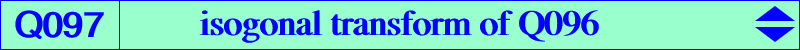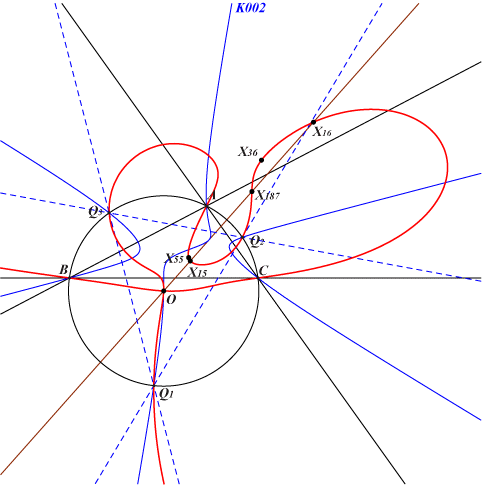too complicated to be written here. Click on the link to download a text file.X(3), X(15), X(16), X(36), X(55), X(187), X(13754) Q1, Q2, Q3 vertices of the Thomson triangle extraversions of X(36), X(55) X(13754) = X(1300)* is the infinite point of the (parallel) lines X(3)X(49), X(4)X(52), X(5)X(389)Q097 is the isogonal transform of the Yiu septic Q096 and the inverse in (O) of Q002. Q097 is a bicircular quintic.